Click to Chat

1800-1023-196

+91-120-4616500

CART 0

• 0

MY CART (5)

Use Coupon: CART20 and get 20% off on all online Study Material

ITEM
DETAILS
MRP
DISCOUNT
FINAL PRICE
Total Price: Rs.

There are no items in this cart.
Continue Shopping• Complete Physics Course - Class 11
• OFFERED PRICE: Rs. 2,968
• View Details

```Gravitational Field and Intensity

The space around a body where the gravitational force exerted by it can be experienced by any other particle is known as the gravitational field of the body. The strength of this gravitational field is referred to as intensity, and it varies from point to point.

Consider the gravitational field of a particle of mass m located at the origin (O).

Suppose that a test particle of mass m0 is placed at the point P(x, y, z). The force of gravitational attraction exerted on the test particle is given by,g = (GMmo/r2)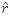where the position vector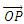= r,

r = OP = || = || = r

and the unit vector,=/ r

The intensity of this gravitational field at a point (P) is given by the force per unit mass on a test particle kept at P, i.e.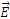=g/mowhereis the gravitational intensity andg  is the gravitational force acting on the mass m0. The gravitational field is, therefore, a vector field.

The gravitational field at P due to a particle of mass m kept at the point O (origin) is given by=g/mo = {-(Gmmo/r2)} * 1/mo = Gm/r2where= xi + yj + zk represents the position vector of the point P with respect to the source at the origin and=/ r represents the unit vector along the radial direction.

The superposition principle extends to gravitational field (intensities) as well:=1 +2 +3 +....+n

where1,2,....n are the gravitational field intensities at a point due to particle 1, 2, ......, n respectively.

For a continuously distributed mass, the formula changes to=∫d, where dgravitational field intensity due to an elementary mass dm.

The gravitational field of a ring on its axis

Let us consider a ring of mass M in the plane perpendicular to the plane of the paper. We want to find the gravitational field on its axis at a distance x.

Consider a differential length of the ring of mass dm.

dE = Fdm/z2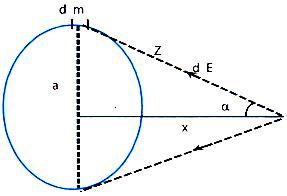The Y-components of the fields due to diametrically opposite elements cancel each other. Thus, the X-components add up.

E = ∫Gdm/z2 cosα = Gcosα/z2 ∫dm = GMcosα/z2 = GMx/(a2+x2)3/2 ←

Field due to a uniform thin spherical shell

Consider a thin spherical shell of radius 'a', mass M and of negligible thickness. Out of the spherical shell we consider a small ring of thickness (R dθ). The shaded ring has mass dm = (M/2) sin θ dθ. The field at P due to thing ring is

dE = Gdm/z2 cosα = GM/2 (sinθ dθ cosα/z2)←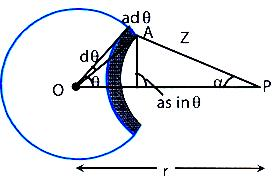From ΔOAP,

z2 = a2 + r2 - 2ar cos θ

or     2z dz = 2ar sin θ dθ

or     sin θ dθ = z dz/ar

Also, from ΔOAP,

a2 = z2 + r2 - 2zr . cos α ; cos α = (z2+r2-a2)/zr

Thus, dE = GM/(4ar2)[1-(a2-r2)/z2]dz

or     ∫dE = GM/(4ar2)[z+(a2-r2)/z2]

Case I:

P is outside the shell, r > a

E = GM/(4ar2 ) [z+(a2-r2)/z2 ](r-a)(r+a) = GM/r2

We see that the shell may be treated as a point particle of the same mass placed at its centre to calculate the gravitational field at an external point.

Case II:

P is outside the shell, r > a

E = GM/(4ar2)[z+(a2-r2)/z2 ](a-r)(a+r) = 0

We see that the field inside a uniform spherical shell is zero.

Gravitational field outside a solid sphere

The sphere can be thought of as composed of many shells from radius = 0 to radius = a.

The point P is at a distance r from the centre of all these concentric shells.

=>     E =  G/r2[ΔM1 + ΔM2 +...]

E =  GM/r2

Gravitational field inside a uniform solid sphere of radius 'R'

To find the field at a point P inside the sphere at a distance r < R form the centre, let us consider a sphere of radius r.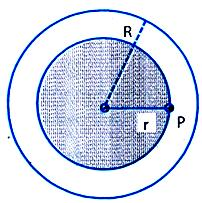Consider a point P on the surface of the shaded sphere. Since this point is inside the shells having radii larger than r, they do not contribute to the field at P. Shells that are less than radius 'r', contribute to the gravitational field at P.

The mass of the sphere of radius r is

M' = (M.4/3 Π r3)/(4/3 Π R3 ) = (Mr3)/R3

.·.     EP = (GM')/r2 = (GM r)/R3

The adjacent graph shows the variation of E due to a solid sphere of radius R with the distance r from its centre.

E =   GM/r2             (r > R)

E =  (GM/R3) r        (r < R)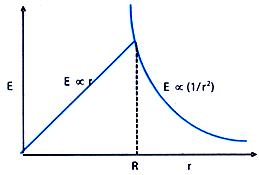This result holds good for the earth if it is assumed to be a uniform solid sphere.

As by definition, g = Fg/m  and also E = Fg/m , so g = E, i.e. acceleration due to gravity and gravitational intensity E at a point are synonymous.

Illustration:

Two concentric shells of masses M1 and M2 are present. Calculate the gravitational force on 'm' due to M1 and M2 at points P, Q and R.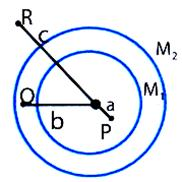Solution:

Field at P, EP = 0      =>     F = 0

Field at Q, due to M2 will be zero but there will be field due to m1,

EQ = (GM1)/b2

=>   F = (GM1m)/b2

Field at R, is the sum of fields due to M1 and M2, ER = G(M1+M2 )/c2

Acceleration due to Gravity (g)

The Earth attracts a mass m on its surface by a force F given as:

F = GMe m/Re2 ,

where Me is the mass of the Earth and Re its radius.

This force imparts an acceleration to the mass m, which is known as acceleration due to gravity (g).

By Newton's second law, acceleration = Force/ Mass ,

=> g = F/m = GMe/Re2

Illustration:  Find the value of g at the surface of Earth?

Radius of Earth = 6.37 × 106 meter.

Mass of Earth = 6 × 1024 kg.

Solution:

As     g = GM/R2                                              ...... (1)

G = 6.67 × 10-11 Nm2 kg-2

M = 6 × 1024 kg

R = 6.37 × 104 m

Put all values in (1), we get g = 9.8 m/s2                                Ans.

Variation of Acceleration due to Gravity (g)

i)       Due to altitude

Consider a mass m at a height h from the surface of the earth. Now, the force acting on the mass due to gravity is F = G, where M is the mass of the earth and R is the radius of the earth.

If the acceleration due to gravity at the given height is g', then mg' = G,

=>     g' = G(Expanding binomially and neglecting the higher order terms).

(ii)    Due to depth:

If a particle of mass m is kept at a depth 'd' form the surface of earth, then gravitational force exerted on the particle of mass 'm'.

(GM' m)/(R-d)2  =  GM(R-d)/R3where M' = mass of earth within radius of (R - d)

.·.      M' = M/R3(R-d)3 = (GM/R3) R(1-d/R) = g(1-d/R)

(iii)  Due to rotation of the earth:

Consider a body at a point with altitude θ, on the surface of the earth.

Let R = radius of the earth and ω = angular velocity of the earth about its own axis.

F.B.D. of the body with respect to an inertial frame is shown in the adjacent figure.Acceleration of the body with respect to the earth's centre O is (Rcos θ)ω2 directed towards the axis of rotation (i.e. the centripetal acceleration).

From Newton's second law in the radial direction

mg - Fn = m(R cos θ) ω2 cos θ

or     Fn = m[g - Rω2 cos2 θ]

or     Fn/m = g' = g - Rω2 cos2 θ

where g' is the apparent value of the acceleration

At poles, θ = 90o => g' = g

At the equator, θ = 0o => g' = g - Rω2

Figure given below has illustrated the variation of g with the distance of separation from Earth's centre.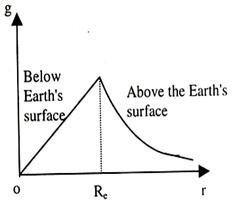Illustration:

Find the value of g at a height equal to the radius of Earth.

Solution:

g = GM/(R+h)2  and g0 = GM/R2  (At the surface of Earth).

g/g0 = R2/(R+h)2 = R2/4R2   ·.·h = R

=> g = g0/4 = 9.8/4 = 2.45 m/s2.                                  Ans.

Caution:    Here h = R, so we cannot apply g' = g(1-2h/Re)

Illustration:

At what angular velocity Earth should rotate, for the weight of an object at the equator to be zero? What would be the duration of a day in this case?

Radius of Earth = 6.4 × 106 m

g0 = 9.8 m/s2

Solution:

For the weight to be zero, the value of g should be zero. That is

Here,     g' = g0 - Reω2 = 0

or ω = √(g0/Re ) = √(9.8/(6.4×106 )) = 1.2 × 10-3 rad/s

The duration of one day will be equal to the time period of rotation

T = 2Π/ω = 2Π/1.2*10-3 sec.

Orbital Velocity

When a satellite revolves in an orbit around a planet, it requires centripetal force to do so. This centripetal force is provided by the gravitational force between the planet and the satellite. If the mass of the satellite is m and that of the planet is M, then the gravitational force between them at a height h above the surface of the planet is

F = GMm/(R+h)2', where R is the radius of the earth.

If the speed of the satellite in its orbit is (R + h), then the required centripetal force is mv2/(R+h).

.·.    mv2/(R+h) = (G Mm)/(R+h)2

or     v = √(GM/(R+h))=√(GM/R(1+h/R)) = √(gR/(1+h/R))

If the height is very small compared to the radius of the earth, then

v = √gR

If the time period of the satellite is 24 hrs rotating in the same sense as the rotation of the earth and if the plane of the orbit is at right angle to the polar axis of the planet (earth), then the satellite will always be above a certain place of the earth.

This kind of a satellite is called geostationary satellite.

Illustration:

An artificial satellite of mass 100 kg is in circular orbit at 500 km above the earth's surface. Take the radius of the earth as 6.5 × 106 m.

(a)    Find the acceleration due to gravity at any point along the satellite path.

(b)    What is the centripetal acceleration of the satellite?

Solution:

Here, h = 500 km = 0.5 × 106 m

R = 6.5 × 106 m

r = R + h = 6.5 × 106 + 0.5 × 106 = 7.0 × 106 m

(a)    g' = g(R/R+h)2  = 9.8((6.5 * 106)/(7.0 * 106))  = 8.45 m/s2

(b)    Centripetal force on the satellite, F = mv2/R

.·.      Centripetal acceleration, a = F/m = v2/r = (√(gR2/r))2/r

= (gR2)/r2 =g R2/(R+h)2 = 8.45 m/s2
```### Course Features

• 101 Video Lectures
• Revision Notes
• Previous Year Papers
• Mind Map
• Study Planner
• NCERT Solutions
• Discussion Forum
• Test paper with Video Solution